## RD Sharma Class 8 Solutions Chapter 1 Rational Numbers Ex 1.5

These Solutions are part of RD Sharma Class 8 Solutions. Here we have given RD Sharma Class 8 Solutions Chapter 1 Rational Numbers Ex 1.5

Other Exercises

Question 1.
Multiply: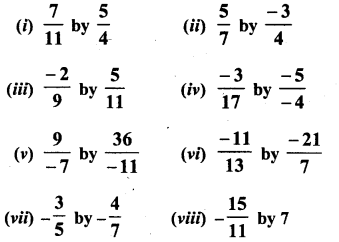Solution:Question 2.
Multiply: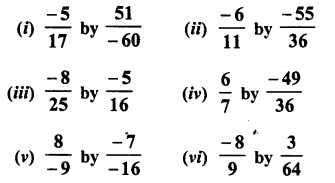Solution: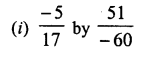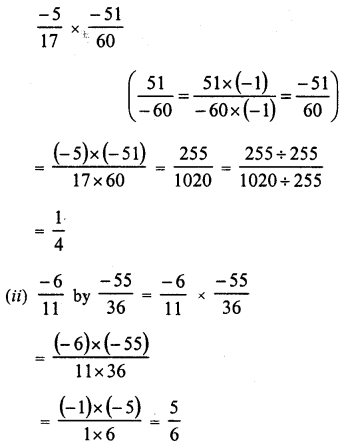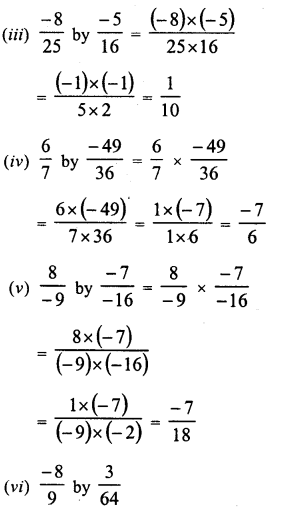Question 3.
Simplify each of the following and express the result as a rational number in standard form :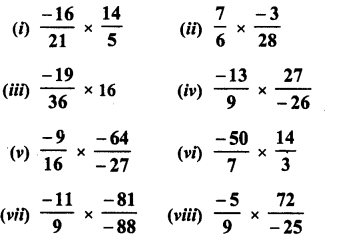Solution: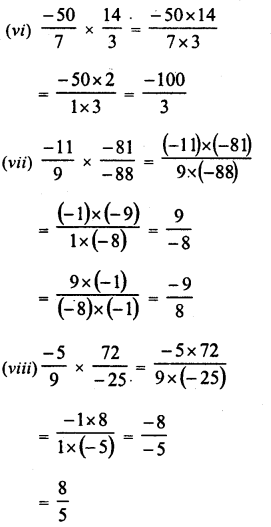Question 4.
Simplify :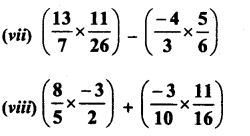Solution: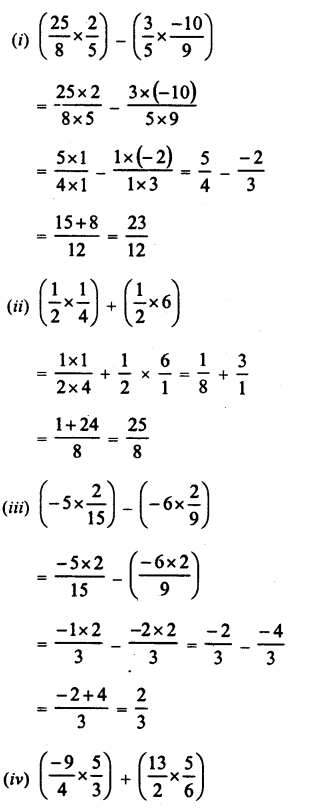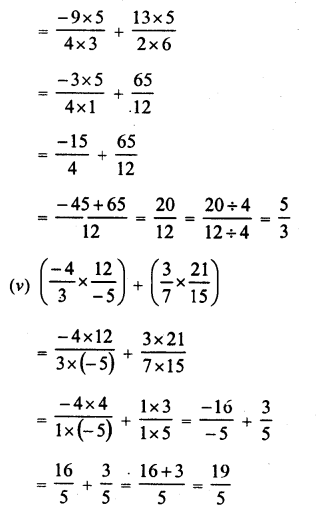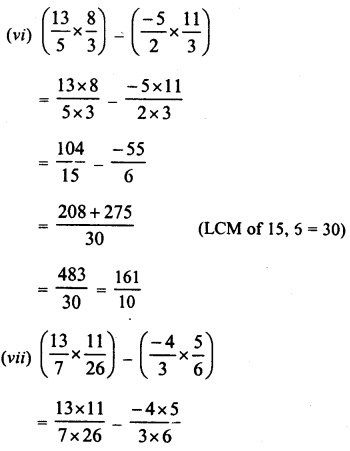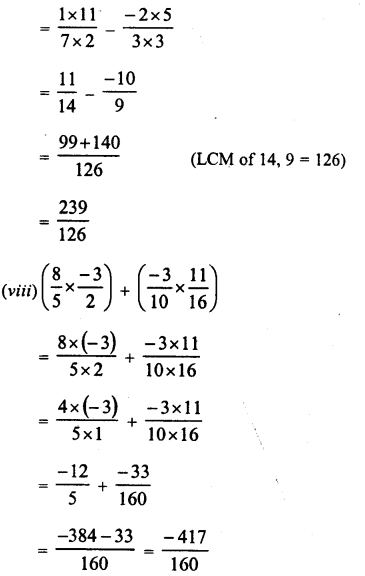Question 5.
Simplify :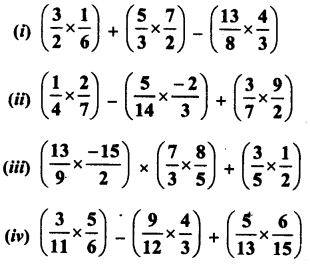Solution: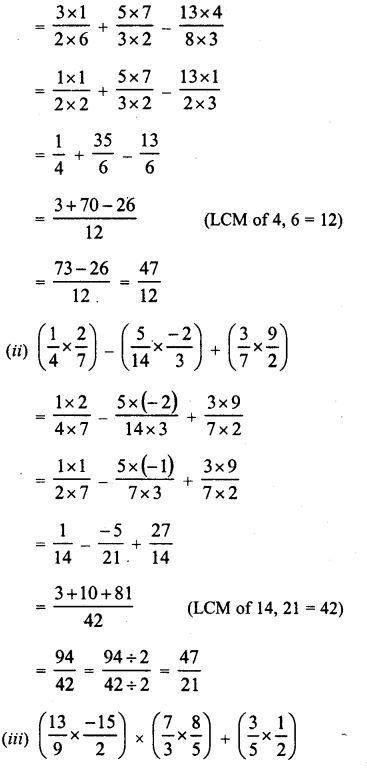Hope given RD Sharma Class 8 Solutions Chapter 1 Rational Numbers Ex 1.5 are helpful to complete your math homework.

If you have any doubts, please comment below. Learn Insta try to provide online math tutoring for you.